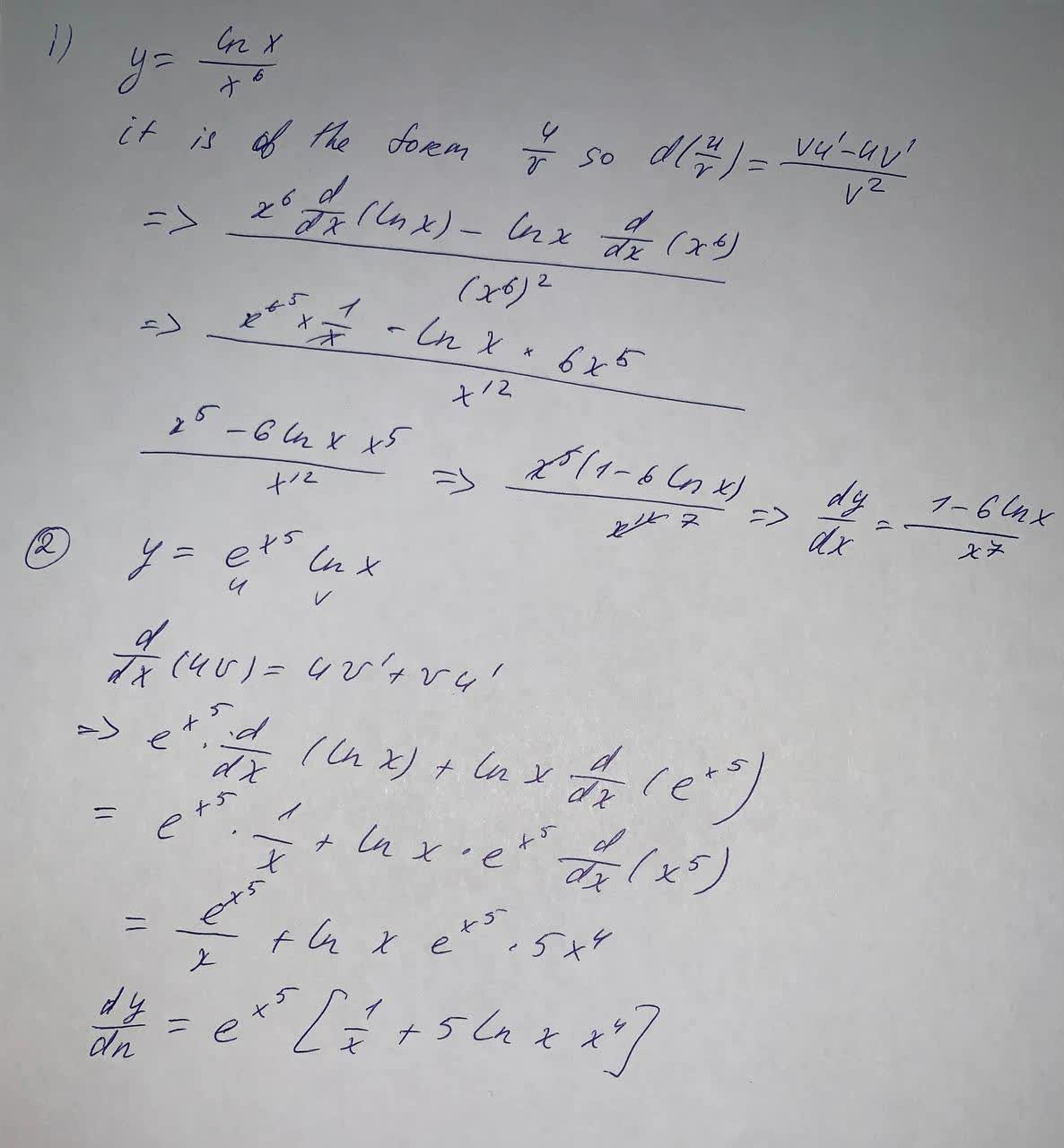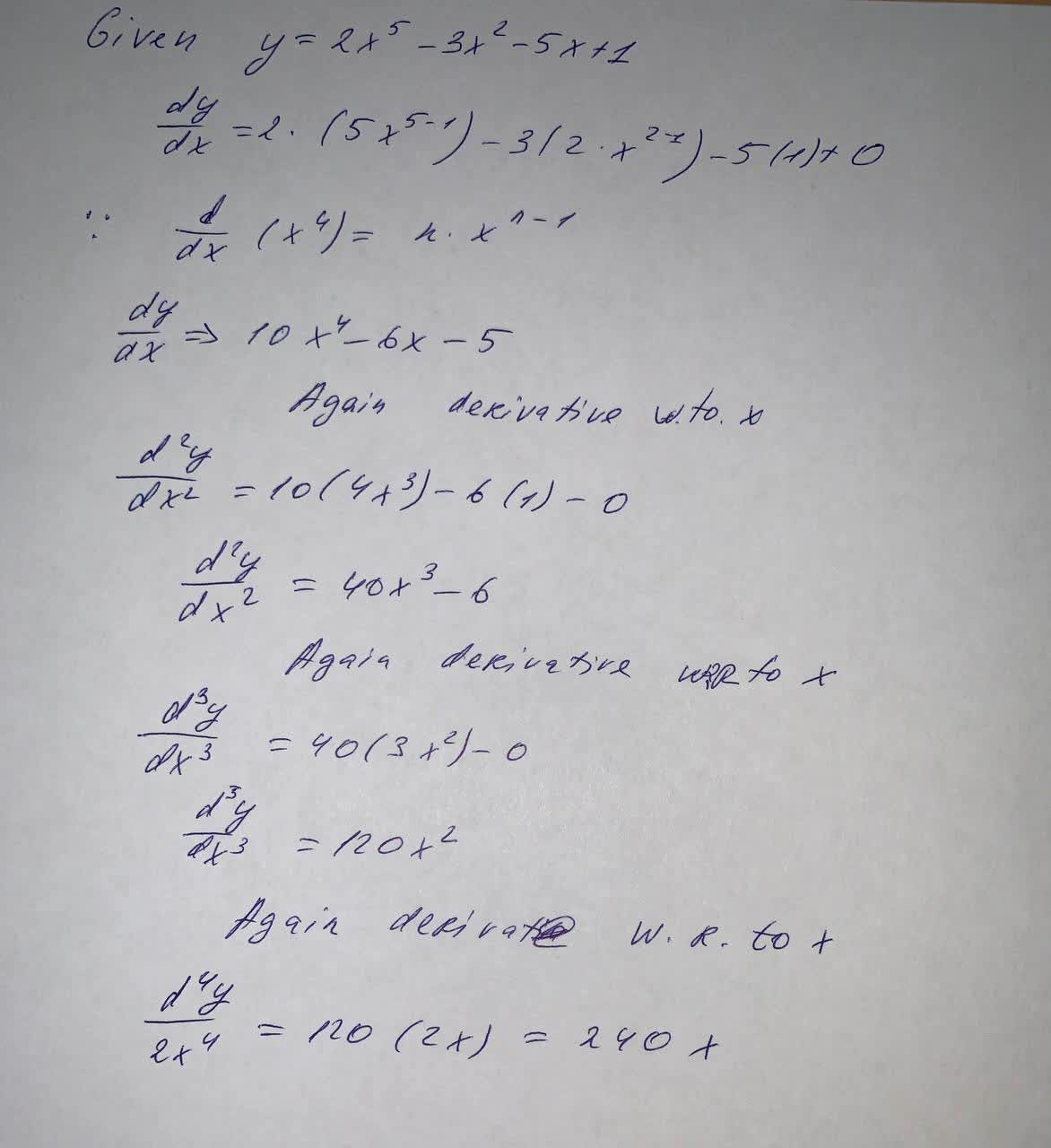#### Didn’t find what you are looking for?

Question# Find the derivative y=\frac{\ln x}{x^6} y=e^{x^5}\ln x Find the indicated derivative of the function. \frac{d^4 y}{dx^4} \text{ of } y=2x^5-3x^2-5x+1

Derivatives
ANSWEREDFind the derivative $$y=\frac{\ln x}{x^6}$$
$$y=e^{x^5}\ln x$$
Find the indicated derivative of the function.
$$\frac{d^4 y}{dx^4} \text{ of } y=2x^5-3x^2-5x+1$$